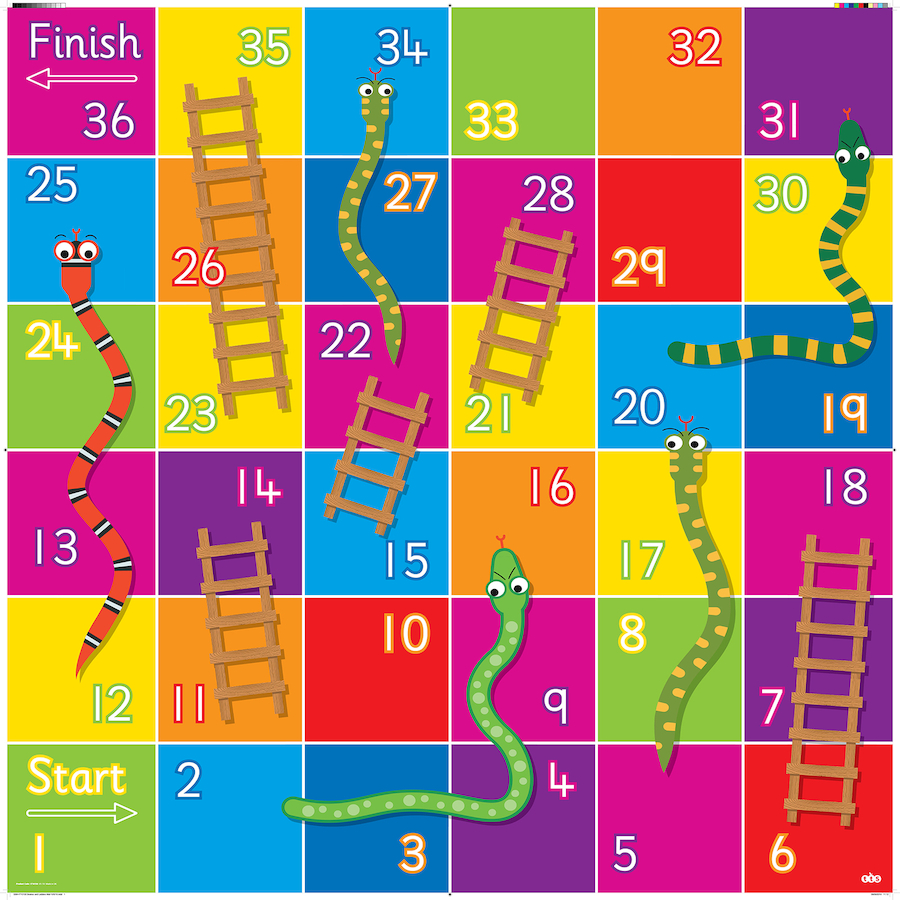• Feedback: We are listening to your every feedback, and taking action to constantly improve your learning experience. If you have any feedback, please use this form: https://thealgorists.com/Feedback.#### Problem Statement:

Cells in the snake and ladder game board are labeled from 1 to n2 in a Boustrophedon style starting from the bottom left of the board and alternating direction each row.
Rules of Snake and Ladder game:
• Start from cell 1.
• Throw the dice and whatever number you get, move on the number of cells on the board.
• If you reach a cell which is base of a ladder, then you have to climb up that ladder without a dice throw.
• If you reach a cell is mouth of the snake, has to go down to the tail of snake without a dice throw.
Follow-up question:
Implement a method that returns the least number of moves required to reach the square n2. If it is not possible to reach the square, return -1.

For the below board the least number of moves required to reach the square with value 36 is 4 by taking below moves:
1 --1st move--> (6 --ladder--> 18) --2nd move-> (15 --ladder-> 22) --3rd move--> (23 --ladder--> 35) --4th move-> 36#### Finding the least number of moves required to reach the finish square:

What we are looking for here is the shortest path from start square (which is 1) to finish square. Every time roll dice we move from one square to another (except of course when the the dice roll tries to lead us to a square greater than the finish square). So these squares could be thought of as nodes in a directed graph. If we are at square i then the child nodes of square i would be (i + 1), (i + 2), (i + 3), (i + 4), (i + 5) and (i + 6).
Weights of all the edges are 1. From the knowledge we have gained from our Algorithms course in the BFS chapter that BFS is an efficient way to find shortest path between source and destination in a graph with all edges with edge-weight equal to one. We have implemented this algorithm in below code. I have discussed in more details in the inline comments in the code below:

#### The above content is written by: